# Implementation of Frequent-Directions Algorithm for Efficient Matrix Sketching

Implement frequent-directions algorithm for efficient matrix sketching. (Edo Liberty (2013) ).

Implementation of Frequent-Directions algorithm for efficient matrix sketching [E. Liberty, SIGKDD2013]

## Example

Here, we use Handwritten digits USPS dataset as sample data. In the following example, we assume that you save the above sample data into `/tmp` directory.

The dataset has 7291 train and 2007 test images in `h5` format. The images are 16*16 grayscale pixels.

### Plot example image

Example the number `8`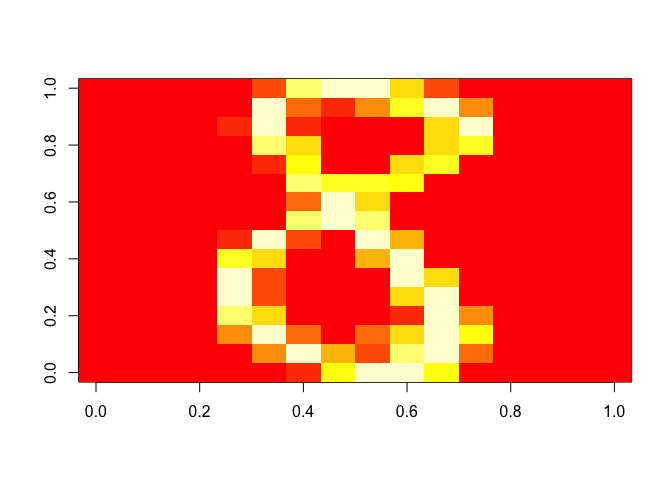### Plot SVD

Plot the original data on the first and second singular vector plane.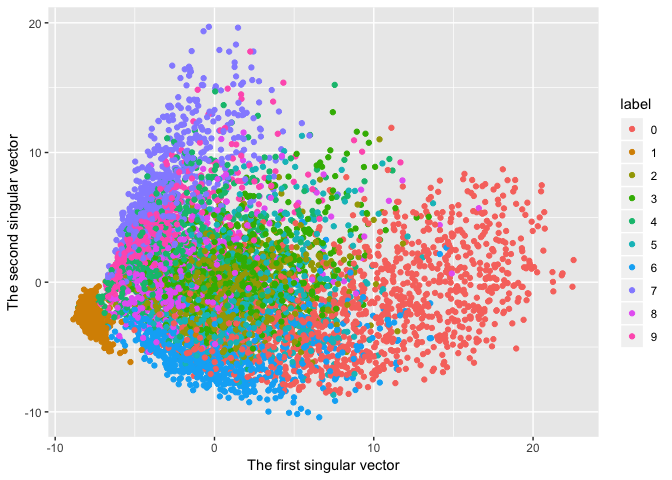### Matrix Sketching

#### l = 8 case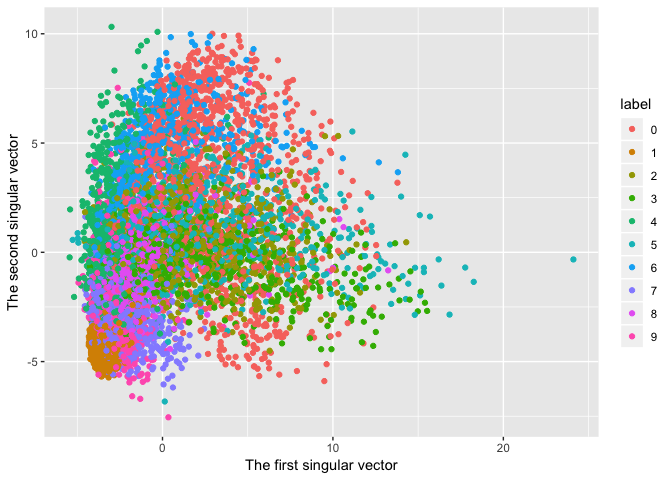#### l = 32 case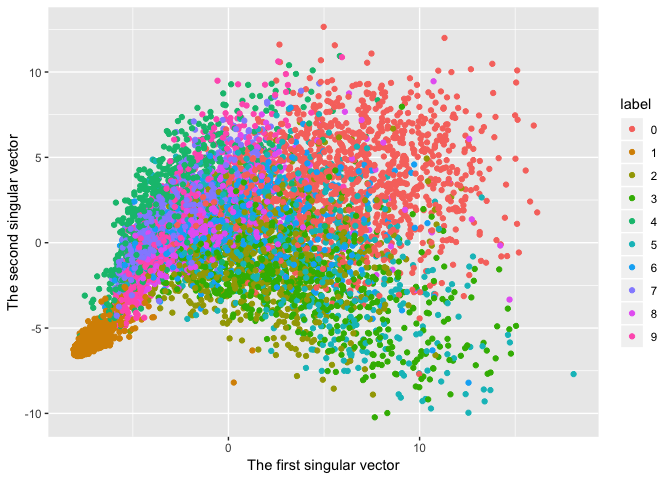#### l = 128 case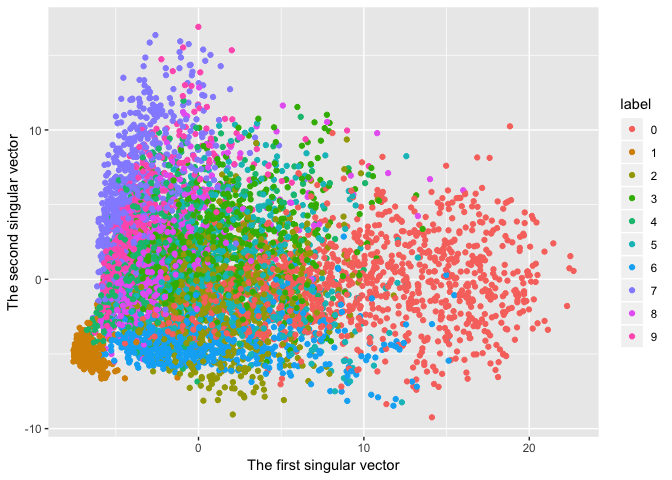This result is almost the same with the original data SVD expression.

That’s why we can think that the original data is expressed with only `128` rows.

# Reference manual

install.packages("frequentdirections")

0.1.0 by Shinichi Takayanagi, a year ago

https://github.com/shinichi-takayanagi/frequentdirections

Browse source code at https://github.com/cran/frequentdirections

Authors: Shinichi Takayanagi [aut, cre] , Nagi Teramo [aut]

Documentation:   PDF Manual